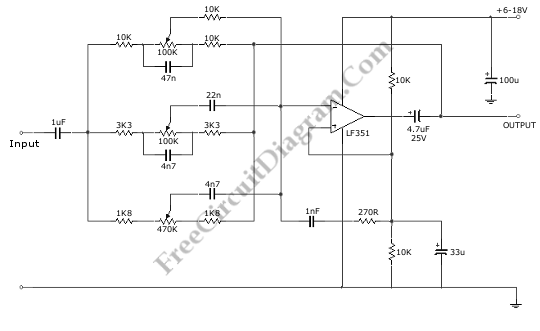# Single Op-Amp 3 Band Equalizer circuitThis is a 3 Band Equalizer circuit. This circuit is made to control the treble, middle and bass range. This circuit uses a single op-amp. With single op-amp we can build equalizer which has three ranges, high frequency, mid frequency, and low frequency. Here is the schematic diagram of the circuit:This circuit has a cut off frequencies of 10kHz, 1kHz, and 50Hz. The boost of this circuit is +/-20dB. This circuit requires a supply voltage from 6V to 30V. Using the maximum supply voltage of 18 Volt, the maximum boost 20dB can be achieved.